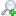# Mathematics (MATH)

MATH 1011  Quantitative ReasoningMATH 1036  Calculus IMATH 1037  Calculus IIMATH 1046  Introductory Linear AlgebraMATH 1056  Discrete Mathematics IMATH 1070  Fundamental Concepts of Mathematics for TeachersMATH 1257  Technical StatisticsMATH 1267  Applied Health StatisticsMATH 1911  Finite MathematicsMATH 1912  Elementary CalculusMATH 1922  Mathematics of Data ManagementMATH 2036  Advanced Calculus IMATH 2037  Advanced Calculus IIMATH 2046  Advanced Linear Algebra IMATH 2056  Discrete Mathematics IIMATH 2076  Probability & Statistics IMATH 2116  GeometryMATH 2216  Introduction to Computational GeometryMATH 2306  History of MathematicsMATH 2386  Problem SolvingMATH 3126  Number TheoryMATH 3127  Combinatorics & Graph TheoryMATH 3136  Real Analysis IMATH 3137  Real Analysis IIMATH 3146  Complex Analysis IMATH 3156  Algebra IMATH 3157  Algebra IIMATH 3166  TopologyMATH 3226  Introduction to Stochastic ProcessesMATH 3256  Operations ResearchMATH 3266  Differential Equations IMATH 3267  Differential Equations IIMATH 3276  Probability and Statistics IIMATH 3286  Mathematics of FinanceMATH 3296  Mathematical ModelingMATH 3396  Selected Topics IMATH 3397  Selected Topics IIMATH 4016  Numerical Methods IIMATH 4036  Measure TheoryMATH 4046  Advanced Applications of Probability and StatisticsMATH 4086  Functional AnalysisMATH 4246  OptimizationMATH 4496  Senior Research Project IMATH 4497  Senior Research Project IIMATH 5036  Measure TheoryMATH 5056  AlgebraMATH 5066  General TopologyMATH 5067  Introduction to Algebraic TopologyMATH 5086  Functional AnalysisMATH 5236  Advanced Numerical MethodsMATH 5237  Computational TopologyMATH 5246  OptimizationMATH 5247  Cryptography and Coding TheoryMATH 5256  Graph TheoryMATH 6001  Major Research Paper MathematicsMATH 6100  Thesis in MathematicsMATH 6101  Selected Topics in Pure MathematicsMATH 6201  Selected Topics in Computational MathematicsMATH 6206  Graduate Seminar in MathematicsMATH 6207  Graduate Seminar in MathematicsNipissing University
100 College Drive, Box 5002, North Bay, ON, Canada  P1B 8L7  Tel: 705.474.3450 | Fax: 705.474.1947 | TTY: 877.688.5507
nuinfo@nipissingu.ca